2.1 firmware gives the ability to control the motor in sinusoidal switching mode with FOC (Field Oriented Control) that previously was not fully supported in all operating modes. Sinusoidal commutation with FOC will lead to a quitter motor operation with less torque ripple. Also, the fact that Speed and Position modes work now based on current control, will have as a result the decreased possibility of current spikes that could lead to high current alarms or controller damage.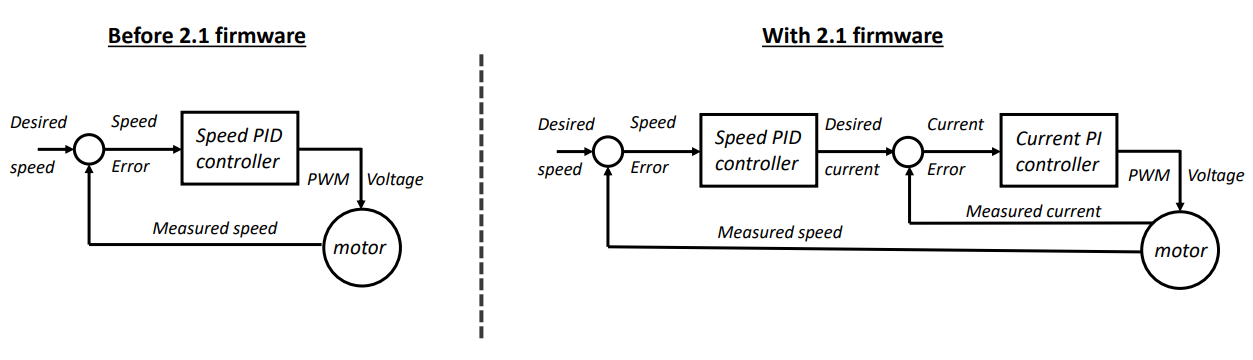1. Migration steps

Because now the FOC, Speed and Position loops will all work cascaded, the PID tuning of each mode should be redone. The FOC mode tuning will be done based on the motor RL parameters and the Speed and Position tuning should be redone from scratch. The gains that will be found to be working will be completely different from the previous ones. If Position mode is not used, only Speed tuning is required, but if Position mode is used, then Speed loop should be tuned as well since Position mode relies on it.

So, the steps that should be followed are:

1. Tune the FOC loop (Configure FOC PI gains)
2. Tune Speed loop (Configure Speed PID gains)
3. Tune Position loop (Configure Position PID gains)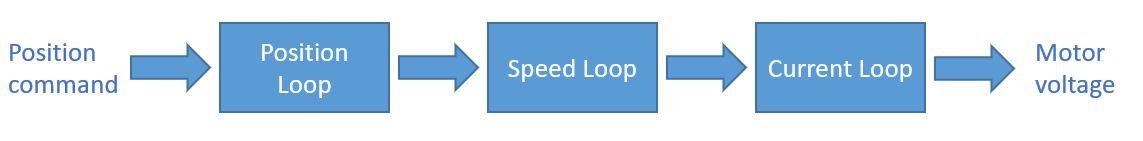2. FOC Loop tuning

Because the tuning of the current loop (FOC) depends only on the parameters of the motor, the FOC gains can be calculated easily, by following a standard formula instead of performing any experimental steps. The only parameters necessary for the calculation are the phase inductance and phase resistance of the motor. With the term "Phase" we mean the value calculated on the motor phase, and not the measured value between two of them (that is called Phase to phase). Please note that if the datasheet does not clarify if the parameters are phase or phase to phase values, then this information should be retrieved from the manufacturer. If the parameters are phase to phase values (p-p) , then the phase value can be derived by dividing them by 2.

The formula to calculate the FOC gains is the following:

FOC Proportional gain = 2 * pi * BW (Hz) * phase inductance (H)

FOC Integral gain = 2 * pi * BW (Hz) * phase resistance (Ohm)

,where pi = 3.14 and inductance and resistance can found on the datasheet. The only parameter needs to be tuned by the user is the Bandwidth (BW) of the FOC loop, in Hz. A value of 50 Hz is the most common configuration for F3 products and it will work sufficiently in most cases. If further, during the tuning of the Speed PID loop, is found that the controller cannot have the desired response and goes into "short error" easily, the Bandwidth value can be decreased. For F3 products typical Badnwidth values are from 10 to 100 Hz.

One other thing to take into consideration when calculating the FOC gains is to input the values in the SI units, that is Henri  and Ohm. So if the datasheet give uH and mOhm, then the respective adjustments should be done.

Example:

Below is a snapshot from the 089LDA30 Nidec motor datasheet: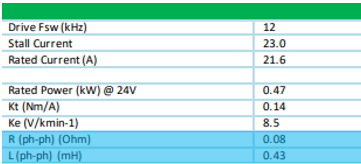The phase-to-phase resistance is 0.08 Ohm, so the phase resistance is 0.04 Ohm

The phase-to-phase inductance is 0.43 mH, so the phase inductance is 0.000215 H

The FOC gains for 50 Hz are calculated as follows:

FOC Proportional gain = 2 * 3.14 * 50 * 0.000215 = 0.06751

FOC Integral gain = 2 * 3.14 * 50 * 0.04 = 12.56

The FOC gains should be configured accordingly for both flux and torque fields.In case the motor specs cannot not be found, 2.1 fw offers a tool called motor characterization that can estimate them. This tool should be used only as a second choice, in case the motor parameters cannot be found from the datasheet. In most of the cases, the motor characterization tool will give a very good approximation.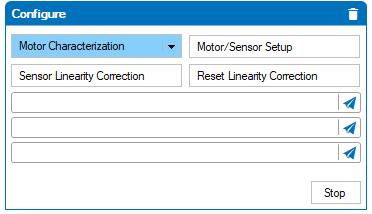The motor characterization will calculate the FOC gains and save them to the controller. Please not that is crucial to configure the nominal motor current on the Reference Power parameter, for the characterization tool to give accurate results.After the gains have been set, the FOC gains should be evaluated n Open Loop by following the next steps:

1. Ensure that by changing the motor command, the flux amps stabilize quickly to zero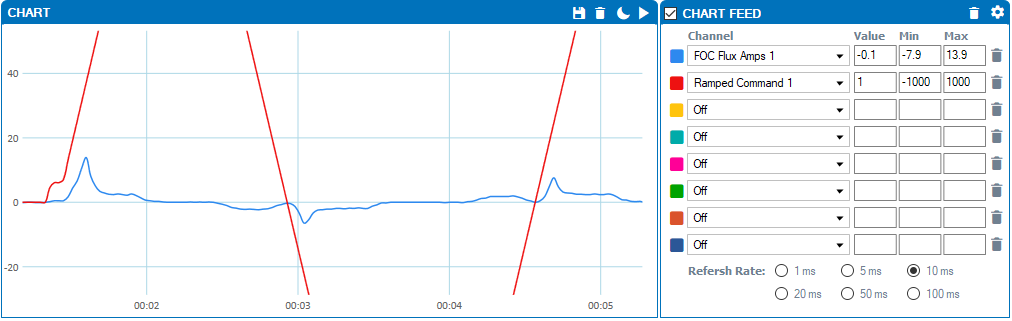2. Give full positive command.  Clear the min/max values and ensure that the flux current is stable and below 1 A. The                 torque amps should be below the motors no load current.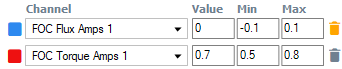3 Monitor the motor speed on the chart and ensure that the speed is symmetrical by giving full positive and negative                 power.If any of the above conditions is not met, then the calculated gains might not be correct (Wrong FOC bandwidth or wrong R-L parameters). Another reason why the FOC control might not be stable is that the R-L parameters are out of range of what the  F3 products can support. See the below table for more information: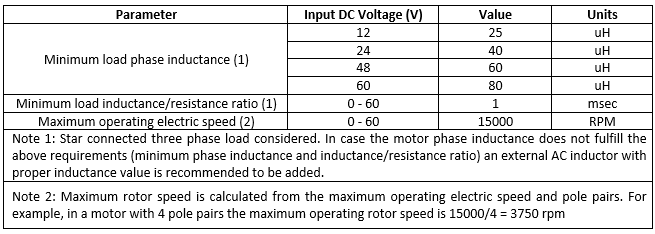To summarize, the steps for the FOC tuning are the following:

• Calculate and set the FOC torque and flux gains
• Evaluate the flux and torque amps in Open loop

3. Speed PID tuning

As mentioned, the Speed PID tuning needs to be re - performed, since the previous PID gains will not work well after the FOC gains have been set (most probably the motor will have many vibraitons). This time, the PID gains are expected to be much smaller than before (might be even ten times smaller) because of the intermediate control loop. Also, the previous usual configuration, where the Integral gain had to be higher than the proportional, is not applied anymore. That way the PID tuning process will be similar to already known PID tuning techniques.

The suggested method to tune the PID loop is following:

• Start with a small value of proportional gain (0.03 should be a good starting value)
• Increase the proportional gain so the speed goes as close to the desired speed as possible without having vibrations.
• Increase the integral gain so the steady state error becomes zero

At this point, the speed response does not need to be very fast. What we want to see here is a nice curve similar to the Open Loop step response of a first order system.The Proportional and integral gains should be finetuned, so the motor response has the previous shape. If the motor overhoots, then the integral gain should be decreased. And if the motor delays to reach the desired speed, then the integral gain should be increased.

The below image gives an example of a tuning, where the Integral term must be increased. This is because the power deriving from the P term stabilizes at point A (when loop error has reached a constan value) and the power provided by the integral term takes time to be developed.Keep in mind that the power provided by the integral part will increase by the time that the error persists, so a higher I gain will make the power increase faster.

Integral power = Sum (Loop error) * Integral gain

In a similar way, if the integral gain is high, the loop error will be accumulated fast and the speed can overshootThe P and I gains should be set that way so they cooperate together to provide a stable response. Once the proper PI gains have been found, they can be increased by the same factor, so the speed of the loop increases without loosing its stability. In ather words by multiplying both Proportional and Integral gains with the same number the Speed Loop bandwidth can be increased.One thing to keep in mind is the existance of ramped command. The controller will never give an input to the PID from from the motor command that the user sends, but it will gradually increase the set point by following the configured acceleration and deceleration. This will be the ramped command.There is no point trying to make the speed exceed the ramped command, or configuring a very high acceleration/deceleration that the motor can't achieve. The proper tuning should be done once the motor can follow closely the given ramped command. If each motor is well tuned and can follow exactly the given command, then the vehicle will be resonsive in all requested scenarios, such as precise turning, following a magnetic tape, performing a safety deceleration etc.

Once the controller is tuned, the speed reponse of each motor should look like below: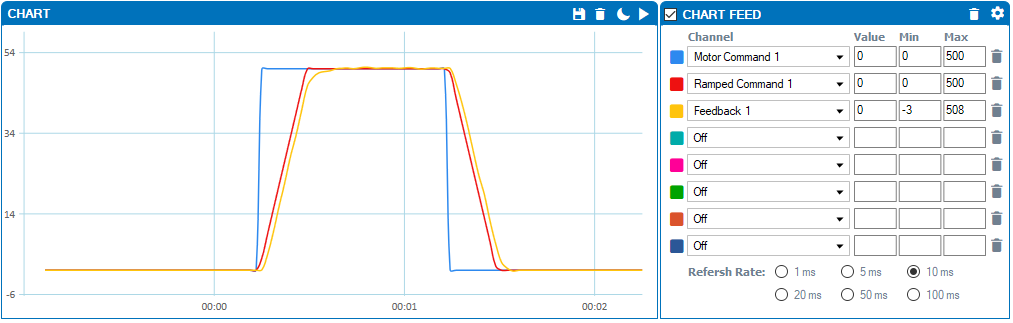Below are some tips for the tuning process:

1. Configure the watchdog timer

SInce in most of the cases the available spece for the tuning will be limited, the wathdog timer of the controller can be used to stop both motors after some time. The job of the wathdog timer is to stop the motor if a new motor command is not received for a cofigured amount of time.

Please notice that in that case the slide bar should be muted, otherwise it will send periodically motor commands and the watchdog timer will never expire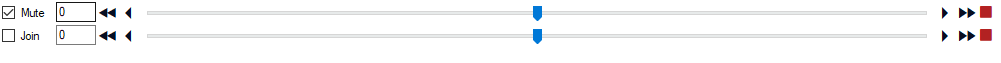One other thing to notice is the fault deceleration. Once the watchdog timer expires, the controller will brake the motors by using the fault deceleration. So this parameter should be set to the desired deceleration of the motor.The wathcdog timer can be configured to a value, like 3 sec.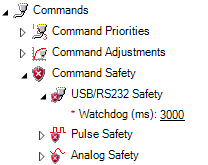Make sure that there is enough time for the motor to reach the set speed befoe decelerating otherwise the speed response will be triangular instead of trapezoidal.

Watchdog timer is 3 sec: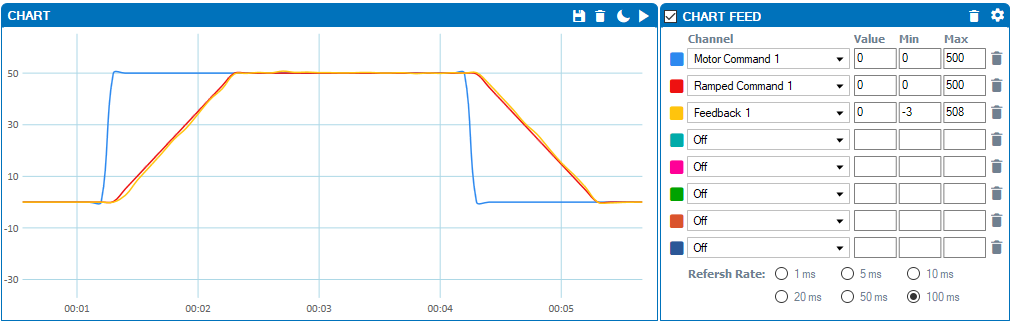Watchdog timer is 1 sec:2. Cofigure the Amps limit

Amps limit parameter must be configured to the peak current of the motor. The controller will not allow the current of the motor to exceed that value, bit this will also decrease the motor performance. Make sure that the amp limit current is not set lower than the current of the motor can handle.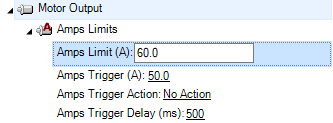3. Use the Run tab chart

Go at the run tab and monitor the Ramped command, feedback, motor amps and motor power for both channelsNote the following:

• Motor amps is not able to exceed tha amps limit value
• If the motor power is saturated (1000) it means that the controller has provided the maximum voltage (100% PWM) and the speed response cannot be increased

4. Use the command boxes

In Closed Loop Speed, the same command can be given in both motors in the following way:

!S cc nn,

where !S is the speed command, cc is the channel and nn the RPM value. Two commands can be sent at the same time using an underscore: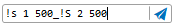That way, the motor command will be given only once, the motor will accelerate to the desired speed and then decelerate to 0 after the watchdog has been expired.

5. Don't forget to adjust the FOC Bandwidth

As metnioned, if the controller goes on short error easily, when it tries to decelerate, then it might help reducing a bit the FOC loop bandwidth (decrease both FOC P and FOC I gains by the same factor).

Previous FOC gains (50 Hz):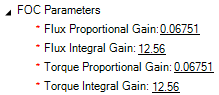Decreased FOC gains (35 Hz):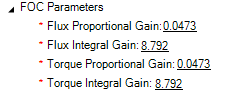6. Adjust the Speed filter Bandwidth

2.1a firmware uses a speed filter that is by default set to 45 Hz. If there are vibrations, the speed filter cut off frequency can be decreased so the high frequency component of the feedback will be off. please note that decreasing the filter bandwidth can affect the rensosivness of the feedback in disturbances.

The command to configure the filter bandwidth is below:

^LPFB cc nn

where cc is the channel and nn the cutoff frequency. Because LPFB is a configuration command, the changes need to be savd to flash memory with the following command:

%EESAV 321654987

e.g.

Chenge the Speed filter bandwidth to 35 Hz. Save configurations to flash memory, so the value is changed after controller reset: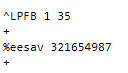Position PID tuning

The position loop can be tuned once the FOC and Speed loop have already been tuned. The position loop will operate by utilizing the speed and position loops. The tuning of the position loop is usually simpler and requires only the addition of proportional gain. Start with a small value (something between 0.01 and 0.1, depending on the resolution of the feedback sensor) and adjust it accordingly. Monitor the loop error and the motor speed to confirm that the motor is following the desired speed and position profile.